JEE  >  Test: MCQs (One or More Correct Option): Electrochemistry | JEE Advanced

# Test: MCQs (One or More Correct Option): Electrochemistry | JEE Advanced

Test Description

## 24 Questions MCQ Test Chemistry 35 Years JEE Main & Advanced Past year Papers | Test: MCQs (One or More Correct Option): Electrochemistry | JEE Advanced

Test: MCQs (One or More Correct Option): Electrochemistry | JEE Advanced for JEE 2023 is part of Chemistry 35 Years JEE Main & Advanced Past year Papers preparation. The Test: MCQs (One or More Correct Option): Electrochemistry | JEE Advanced questions and answers have been prepared according to the JEE exam syllabus.The Test: MCQs (One or More Correct Option): Electrochemistry | JEE Advanced MCQs are made for JEE 2023 Exam. Find important definitions, questions, notes, meanings, examples, exercises, MCQs and online tests for Test: MCQs (One or More Correct Option): Electrochemistry | JEE Advanced below.
 1 Crore+ students have signed up on EduRev. Have you?
Test: MCQs (One or More Correct Option): Electrochemistry | JEE Advanced - Question 1

### The standard reduction potentials at 298 K for the following half reactions are given against each              (1981 - 1 Mark)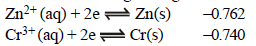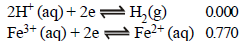which is the strongest reducing agent?

Detailed Solution for Test: MCQs (One or More Correct Option): Electrochemistry | JEE Advanced - Question 1

More negative is the value of reduction potential, higher will be the reducing property, i.e., the power to give up electrons.

Test: MCQs (One or More Correct Option): Electrochemistry | JEE Advanced - Question 2

### Faraday’s laws of electrolysis are related to the          (1983 - 1 Mark)

Detailed Solution for Test: MCQs (One or More Correct Option): Electrochemistry | JEE Advanced - Question 2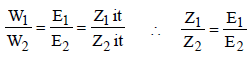Test: MCQs (One or More Correct Option): Electrochemistry | JEE Advanced - Question 3

### A solution containing one mole per litre of each Cu(NO3)2; AgNO3; Hg2(NO3)2; is being electrolysed by using inert electrodes. The values of standard electrode potentials in volts (reduction potentials) are : (1984 - 1 Mark)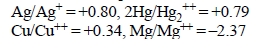With increasing voltage, the sequence of deposition of metalson the cathode will be :

Detailed Solution for Test: MCQs (One or More Correct Option): Electrochemistry | JEE Advanced - Question 3

The reduction potentials (as given) of the ions are in the order :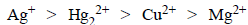Mg2+ (aq.) will not be reduced as its reduction potential is much lower than that of water (–0.83 V). Hence the sequence of deposition of the metals will be Ag, Hg, Cu.

Test: MCQs (One or More Correct Option): Electrochemistry | JEE Advanced - Question 4

The electric charge for electrode deposition of one gramequivalent of a substance is : (1984 - 1 Mark)

Detailed Solution for Test: MCQs (One or More Correct Option): Electrochemistry | JEE Advanced - Question 4

Charge of one mole of electrons = 96500 C ∴ 1 mole gram equivalent of substance will be deposited by one mole of electrons.

Test: MCQs (One or More Correct Option): Electrochemistry | JEE Advanced - Question 5

The reaction :
½ Hg2(g) + AgCl(s)  →  H+(aq) + Cl(aq) + Ag(s)  occurs in the galvanic cell

Detailed Solution for Test: MCQs (One or More Correct Option): Electrochemistry | JEE Advanced - Question 5

NOTE : Oxidation is loss of electron and in a galvanic cell it occurs at anode. Reduction is gain of electron and in a galvanic cell it occurs at cathode.
Cell representation :
Anode / Anodic electrolyte || Cathodic electrolyte / Cathode
Reaction at Anode : H2 → 2H+ + 2e
Reaction at Cathode : AgCl + e → Ag + Cl

Test: MCQs (One or More Correct Option): Electrochemistry | JEE Advanced - Question 6

A solution of sodium sulphate in water is electrolysed usinginert electrodes. The products at the cathode and anode arerespectively (1987 - 1 Mark)

Detailed Solution for Test: MCQs (One or More Correct Option): Electrochemistry | JEE Advanced - Question 6

Water is reduced at the cathode and oxidized at the anode instead of Na+ and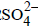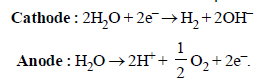Test: MCQs (One or More Correct Option): Electrochemistry | JEE Advanced - Question 7

The standard oxidation potentials, Eº, for the half reactions are as           (1988 - 1 Mark)
Zn = Zn2+ + 2e; Eº = +0.76 V
Fe = Fe2+ + 2e; Eº = +0.41 V
The EMF for the cell reaction :
Fe2+ + Zn →   Zn2+ + Fe

Detailed Solution for Test: MCQs (One or More Correct Option): Electrochemistry | JEE Advanced - Question 7

TIPS/FORMULAE :
(i) In a galvanic cell oxidation occurs at anode and reduction occurs at cathode.
(ii) Oxidation occurs at electrode having higher oxidation potential and it behaves as anode and other electrode acts as cathode.
(iii) ECell = EC – EA
(substitute reduction potential at both places).

Fe2+ + Zn →  Zn2+ + Fe
∵ Zn →  Zn++ + 2e- and Fe2+ + 2e- →  Fe
∴ Zn is anode and Fe is cathode.
Ecell = EC – EA = –0.41 – (–0.76) = 0.35V.

Test: MCQs (One or More Correct Option): Electrochemistry | JEE Advanced - Question 8

A dilute aqueous solution of Na2SO4 is electrolyzed using platinum electrodes.The products at the anode and cathode
are:

Detailed Solution for Test: MCQs (One or More Correct Option): Electrochemistry | JEE Advanced - Question 8

H2O is more readily reduced at cathode than Na+. It is also more readily oxidized at anode than. Hence, the electrode reactions are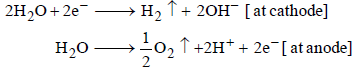Test: MCQs (One or More Correct Option): Electrochemistry | JEE Advanced - Question 9

The standard reduction potentials of Cu2+ | Cu and Cu2+|Cu+ are 0.337 V and 0.153 respectively. The standardelectrode potential of Cu+ |Cu half cell is (1997 - 1 Mark)

Detailed Solution for Test: MCQs (One or More Correct Option): Electrochemistry | JEE Advanced - Question 9

We have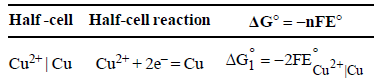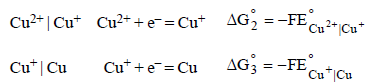From the half-cell reactions, it follows that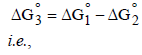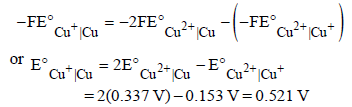Test: MCQs (One or More Correct Option): Electrochemistry | JEE Advanced - Question 10

A gas X at 1 atm is bubbled through a solution containing amixture of 1 M Y and M Z at 25°C. If the reduction potentialof Z > Y > X, then, (1999 - 2 Marks)

Detailed Solution for Test: MCQs (One or More Correct Option): Electrochemistry | JEE Advanced - Question 10

The given order of reduction potentials is Z > Y > X. A
spontaneous reaction will have the following
characteristics
Z reduced and Y oxidised
Z reduced and X oxidised
Y reduced and X oxidised
Hence, Y will oxidise X and not Z.

Test: MCQs (One or More Correct Option): Electrochemistry | JEE Advanced - Question 11

For the electrochemical cell, M|M+ || X- | X,E°M+/M =0.44V and E°(X/X) = 0.33V.From this data one can deduce that (2000S)

Detailed Solution for Test: MCQs (One or More Correct Option): Electrochemistry | JEE Advanced - Question 11

For M+ + X → M + X,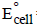= 0.44 – 0.33 = 0.11V is positive, hence reaction is spontaneous

Test: MCQs (One or More Correct Option): Electrochemistry | JEE Advanced - Question 12

Saturated solution of KNO3 is used to make ‘salt-bridge’because (2001S)

Detailed Solution for Test: MCQs (One or More Correct Option): Electrochemistry | JEE Advanced - Question 12

The salt used to make ‘salt-bridge’ must be such that the ionic mobility of cation and anion are of comparable order so that they can keep the anode and cathode half cells neutral at all times. KNO3 is used becasue velocities of K+ and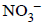ions are nearly same

Test: MCQs (One or More Correct Option): Electrochemistry | JEE Advanced - Question 13

The correct order of equivalent conductance at infinitedilution of LiCl, NaCl and KCl is (2001S)

Detailed Solution for Test: MCQs (One or More Correct Option): Electrochemistry | JEE Advanced - Question 13

As we go down the group 1 (i.e. from Li+ to K+), the ionic radius increases, degree of solvation decreases and hence effective size decreases resulting in increase in ionic mobility.Hence equivalent conductance at
infinite dilution increases in the same order.

Test: MCQs (One or More Correct Option): Electrochemistry | JEE Advanced - Question 14

Standard electrode potential data are useful for understanding the suitability of an oxidant in a redox titration. Some half cell reactions and their standard potentials are given below :                    (2002S)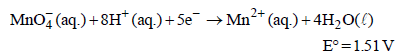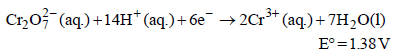Fe3+ (aq.) + e- → Fe2+ (aq.)                      E° = 0.77 V
Cl2(g) + 2e- → 2Cl- (aq.)                           E° = 1.40 V

Identify the only incorrect statement regarding the quantitative estimation of aqueous Fe(NO3)2

Detailed Solution for Test: MCQs (One or More Correct Option): Electrochemistry | JEE Advanced - Question 14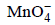will oxidise Cl ion according to the following equation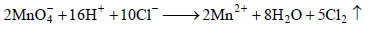The cell corresponding to this reaction is as follows :

Pt, Cl2 (1 atm) | Cl ||, Mn2+, H+ | Pt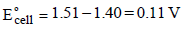being +ve, ΔG° will be -ve and hence the above reaction is feasible.will not only oxidise Fe2+ ion but also Cl ion simultaneously. So the quantitative estimation of aq Fe(NO3)2 cannot be done by this.

Test: MCQs (One or More Correct Option): Electrochemistry | JEE Advanced - Question 15

In the electrolytic cell, flow of electrons is from                (2003S)

Detailed Solution for Test: MCQs (One or More Correct Option): Electrochemistry | JEE Advanced - Question 15

NOTE : In an electrolytic cell, electrons do not flow themselves. It is the migration of ions towards oppositely charged electrodes that indirectly constitutes the flow of electrons from cathode to anode through internal supply.

Test: MCQs (One or More Correct Option): Electrochemistry | JEE Advanced - Question 16

The emf of the cell Zn | Zn2+ (0.01 M) | | Fe2+ (0.001 M) | Fe  at 298 K is 0.2905 then the value of equilibrium constant for
the cell reaction is                      (2004S)

Detailed Solution for Test: MCQs (One or More Correct Option): Electrochemistry | JEE Advanced - Question 16

TIPS/FORMULAE :
Use Nernst's equation;
Cell reaction : Zn + Fe2+ → Zn2+ + Fe
Using Nernst equation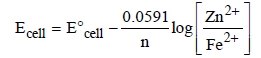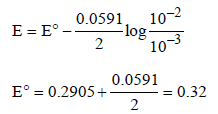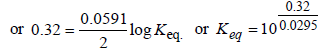Test: MCQs (One or More Correct Option): Electrochemistry | JEE Advanced - Question 17

The rusting of iron takes place as follows                 (2005S)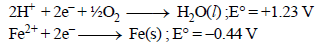Calculate ΔG° for the net process

Detailed Solution for Test: MCQs (One or More Correct Option): Electrochemistry | JEE Advanced - Question 17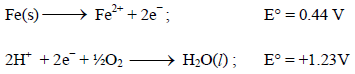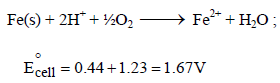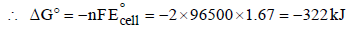Test: MCQs (One or More Correct Option): Electrochemistry | JEE Advanced - Question 18

Electrolysis of dilute aqueous NaCl solution was carriedout by passing 10 milli ampere current. The time required toliberate 0.01 mol of H2 gas at the cathode is (1 Faraday =96500 C mol–1)              (2008S)

Detailed Solution for Test: MCQs (One or More Correct Option): Electrochemistry | JEE Advanced - Question 18

Give : I = 10 milliamperes ; IF = 96500 C mol–1
t = ? ; Moles of H2 produces = 0.01 mol
From the law of electrolysis, we have
Equivalents of H2 produces =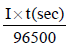Substituting given values, we get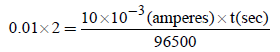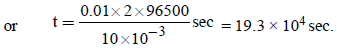i.e. (b) is the correct answer

Test: MCQs (One or More Correct Option): Electrochemistry | JEE Advanced - Question 19

AgNO3(aq.) was added to an aqueous KCl solution gradually and the conductivity of the solution was measured.
The plot of conductance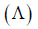versus the volume of AgNO3  is             (2011)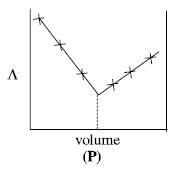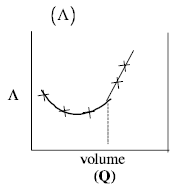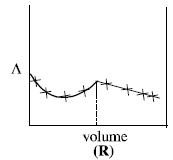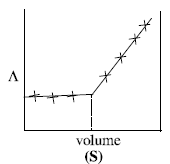Detailed Solution for Test: MCQs (One or More Correct Option): Electrochemistry | JEE Advanced - Question 19

AgNO3 (aq) + KCl(aq)  →  AgCl(s) + KNO3(aq)
Conductivity of the solution is almost compensated due to formation of KNO3(aq). However, after at end point, conductivity increases more rapidly due to addition of excess AgNO3 solution.

Test: MCQs (One or More Correct Option): Electrochemistry | JEE Advanced - Question 20

Consider the following cell reaction:               (2011)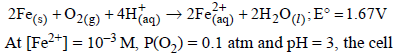potential at 25ºC is

Detailed Solution for Test: MCQs (One or More Correct Option): Electrochemistry | JEE Advanced - Question 20

Here n = 4, and [H+] = 10–pH = 10–3
Applying Nernst equation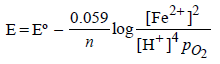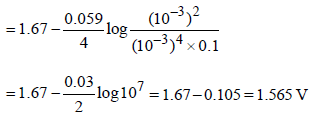Test: MCQs (One or More Correct Option): Electrochemistry | JEE Advanced - Question 21

For the following electrochemical cell at 298 K, Pt(s)|H2(g, 1 bar)| H+ (aq, 1 M) || M4+ (aq), M2+ (aq)|Pt(s)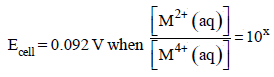Given :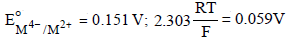The value of x is                                (JEE Adv. 2016)

Detailed Solution for Test: MCQs (One or More Correct Option): Electrochemistry | JEE Advanced - Question 21

At anode : H2(g)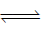2H+ (aq) + 2e
At cathode : M4+ (aq) + 2eM2+ (aq)
Net cell reaction : H2(g) + M4+ (aq)2H+ (aq) + M2+ (aq)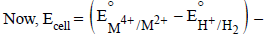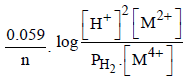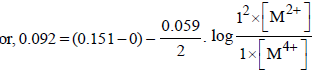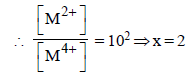Test: MCQs (One or More Correct Option): Electrochemistry | JEE Advanced - Question 22

The standard reduction potential values of three metalliccations, X, Y and Z are 0.52,– 3.03 and – 1.18 V respectively.The order of reducing power of the corresponding metals is              (1998 - 2 Marks)

Detailed Solution for Test: MCQs (One or More Correct Option): Electrochemistry | JEE Advanced - Question 22

NOTE : More negative or lower is the reduction potential , more is the reducing property. Thus the reducing power of the corresponding metal will follow the reverse order, i.e. Y > Z > X.

*Multiple options can be correct
Test: MCQs (One or More Correct Option): Electrochemistry | JEE Advanced - Question 23

For the reduction of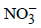ion in an aqueous solution, E° is + 0.96V. Values of E° for some metal ions are given below
V2+ (aq) + 2e–  → V E° = – 1.19 V
Fe3+ (aq) + 3e → Fe E° = – 0.04 V
Au3+ (aq) + 3e → Au E° = + 1.40 V
Hg2+ (aq) + 2e →Hg E° = + 0.86 V

The pair(s) of metals that is(are) oxidized byinaqueous solution is(are) (2009)

Detailed Solution for Test: MCQs (One or More Correct Option): Electrochemistry | JEE Advanced - Question 23

The species having less reduction potential with respect to(E° = + 0.96 V) will be oxidised by. These species are V, Fe and Hg.

Test: MCQs (One or More Correct Option): Electrochemistry | JEE Advanced - Question 24

In a galvanic cell, the salt bridge              (JEE Adv. 2014)

Detailed Solution for Test: MCQs (One or More Correct Option): Electrochemistry | JEE Advanced - Question 24

Salt bridge is introduced to keep the solutions of two electrodes separate, so that the ions in electrodes do not mix freely with each other. Salt bridge maintains the diffusion of ions from one electrode to another.

## Chemistry 35 Years JEE Main & Advanced Past year Papers

142 docs|66 tests
 Use Code STAYHOME200 and get INR 200 additional OFF Use Coupon Code
Information about Test: MCQs (One or More Correct Option): Electrochemistry | JEE Advanced Page
In this test you can find the Exam questions for Test: MCQs (One or More Correct Option): Electrochemistry | JEE Advanced solved & explained in the simplest way possible. Besides giving Questions and answers for Test: MCQs (One or More Correct Option): Electrochemistry | JEE Advanced, EduRev gives you an ample number of Online tests for practice

## Chemistry 35 Years JEE Main & Advanced Past year Papers

142 docs|66 tests